# Vector in game development: Understand the basics of vector math

You know this moment would come, it’s time to speak about math. But what you’re going to see here is the basic math used for the development of video games, to be more specific, we’re going to see how to use vector in game development and all the operations around this “math” instrument.

In fact, without a certain knowledge of the mathematical field and how math can be used to develop your games, it’s difficult to solve some problems in our games.

I know that most of the game engine like Unity or Unreal already has a physics or graphics engine that solve most of the problems. But still, you need to know the mathematical tools needed to interact with the game world.

In this first post, you’re going to see the basic entity that you’ll probably find most in any games that you’re going to develop, the Vector.

## The vector in game development:

Vector is a quantity that has both magnitude and direction in an n-dimensional space.

We can basically see it as an arrow: ———>.

The arrow’s length is its magnitude and the tip of the arrow indicate the direction of the vector itself.

### Ok, but what can a vector be useful for?

Vectors can be used to describe all sorts of physics situations and more. In fact, a ball that is kicked in a football game can be a situation where vectors are used to describe the force applied to the ball along with the direction where the force is applied. Same goes for a bullet shot from a gun.

Have you ever played the game Angry Birds? The force and the direction applied to the bird can also be described using vectors. And what about the velocity of a car running in the last amazing racing game that we built? That also can be described using vectors. And the list goes on.

### How is a vector defined in the game engine?

In most game engines, you’ll find a vector as a description of its 3 components X, Y, Z.

Why 3 components? Because our game is defined in a 3D world so when we apply a force to a game object in our engine, we can apply it in each arbitrary direction inside the game (unless there are restrictions).

For example if we want to describe an elevator that is moving up and down in our Unity game, our vector we’ll be formed by these coordinates (X = 0, Y = velocity of the elevator, Z = 0).

This is the definition of a vector in Unity C#:

Vector3 aVector = new Vector3(0,3,10)

### Scalar vector

A scalar vector is nothing more than the magnitude representation of the vector and is usually written in italics ( e.g v) while vectors are written in boldface ( e.g., v).

### Use vector to represent a point in space

Image we want to describe where an object is placed in our 3D or 2D game, how can we do that? Taken the origin of our 3D world, we can draw a vector from it to the game object and this is the vector that describes the position of the game object inside the game world.

In fact, imagine drawing an “arrow” from the origin of the 3D world to the position of the game object, that is the exact vector that we’re looking for.If you look at the image you’ll notice that the vector in question is nothing more than the coordinate of X,Y,Z in a 3D cartesian system which in our case represent the game world space.

So we understand that vectors can not only be used to describe physical simulations, like the motion of a rigid body in a 3D world, but they can also be helpful to describe the position of an object inside the game world.

## Orientation of vectors

### Left-hand system vs right-hand system

In the right-hand system (RH), when curling your fingers around the z-axis with the thumb pointing toward the z positive direction, your fingers point from the x-axis toward the y-axis.

In the left system, it is the same method but using the left hand.It’s important to know which system your engine is using and stick to that convention when using it.

## Vector operations

Let’s take a look at some of the basic operations that we can do with vectors and how they can be used inside your games.

### Multiplications by a scalar

Multiplication by a scalar has the effect to change the magnitude of the vector.  We simply multiply each component of the vector with the scalar value.

For example, the vector A: (2x, 3y,5z) multiplied by 2 = A * 2 = (4x,6x,10z)

Adding and subtracting vectors are quite easy Given two vectors A and B, the addition of the 2 is the sum of the single components of the vector.

For example :

a + b = [(ax + bx), (ay + by), (az + bx)].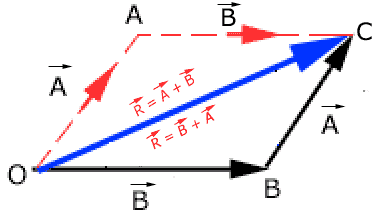Image from citycollegiate

Same things goes with the subtraction.

a + b = [(ax – bx), (ay – by), (az – bx)].

A good use of the addition and subtraction of vectors in games could be in finding the distance between 2 game objects.In this case, the distance from A to B is defined as the sum of the 2 vectors (A + B).

### Normal vector

A vector is said to be normal to a surface if it is perpendicular to that surface.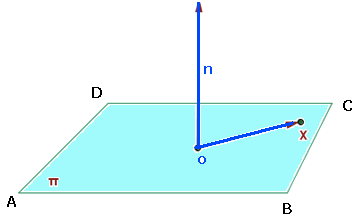### Normalized vector

It is a simple vector that has a magnitude of length equals to one unit (don’t confuse it with the normal vector).

### Dot.productThe Dot Product can be helpful when finding the angle between 2 vectors and by consequence, if the 2 vectors are perpendicular to each other. If the dot product is zero, then the 2 vectors are perpendicular.

Example of the results of some dot products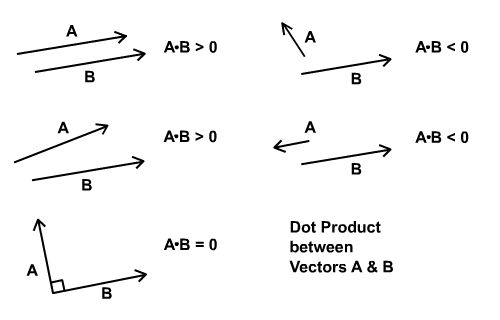Image from: cdn.tutsplus.com

It can also be done by:

A dot B = |A| * |B| * cos “angle between”

Another example usage of the dot product could be when calculating the speed at which a character/game object is moving in the direction of a slope. Other examples include finding how much of a character’s velocity is in the direction of gravity, finding how long until a movement crosses a threshold, and more.

### Cross.product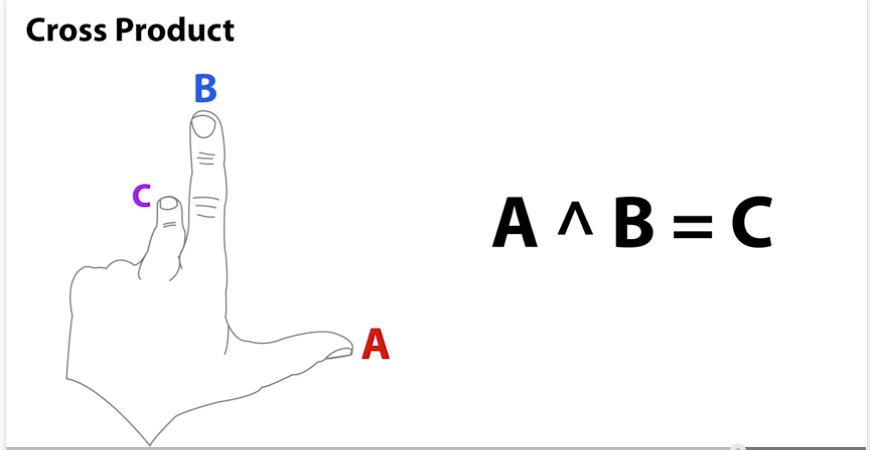Example:With the cross product, we can find C, which is the axes where we can rotate the tank in our game.

Magnitude of the cross product: |a x b| = |a|  |b| sin (angle between a and b).

The direction of the cross product: Use the right-hand rule to determine the direction.

NB: Unity, can perform all of these operations without having to calculate them manually. But it is just helpful to know how they work and where to use them.

### LINEAR INTERPOLATION (LERP)

The linear interpolation (LERP) is one of the most common operations used in game development.

For example, if we want to smoothly animate from point A to point B over the course of two seconds at 30 frames per seconds, we would need to find 60 intermediate positions between A and B.

A linear interpolation is a mathematical operation to find an intermediate point between two known points. The operation can be defined as follows: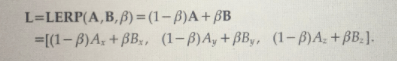Where β is a value between 0 and 1 where 0 represents the initial position of the Lerp and 1 as the final position.

As you can see from the definition, we’re representing the lerp as a Vector.

Here is an example to visualize the lerp in action: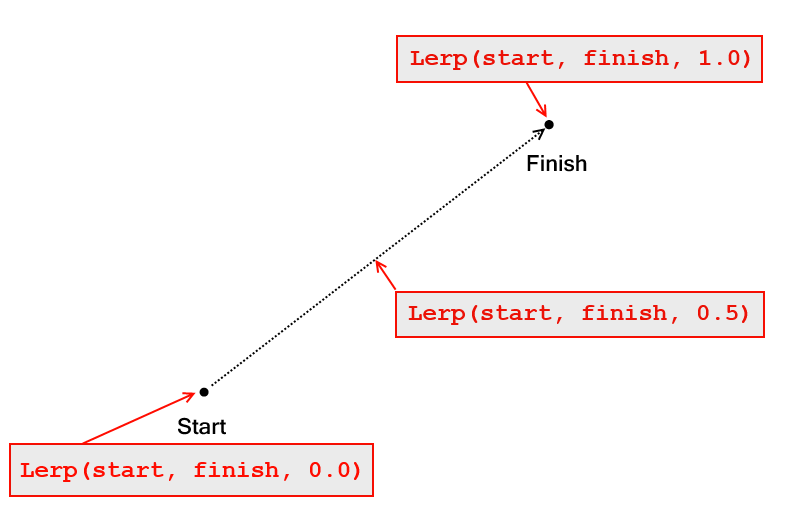Most game engines has a lerp function ready to be used. Here is an example of a code using the lerp function in Unity:

```using UnityEngine;
using System.Collections;
public class ExampleClass : MonoBehaviour {
public Vector3 startPosition;
public Vector3 endPosition;
public float speed = 1.0F;
private float startTime;
private float journeyLength;
void Start() {
startTime = Time.time;
journeyLength = Vector3.Distance(startPosition, endPosition);
}
void Update() {
float distCovered = (Time.time - startTime) * speed;
float fracJourney = distCovered / journeyLength;
transform.position = Vector3.Lerp(startPosition,endPosition, fracJourney);
}
}
```

As you can see, we’ve used vectors to describe the start position and the end position of the lerp. The Vector3.Lerp is a function from Unity that executes the LERP operation for you.

## Use vectors to describe objects and interactions in the game world.

### Model space

The model space is the reference system used by the object in question.

For example, a character game object’s forward axis points in the direction that the object is naturally facing.

Up: is the direction that point towards the top of the object

The left or right are the axes that points to its left or right sides respectively.

Example with a plane: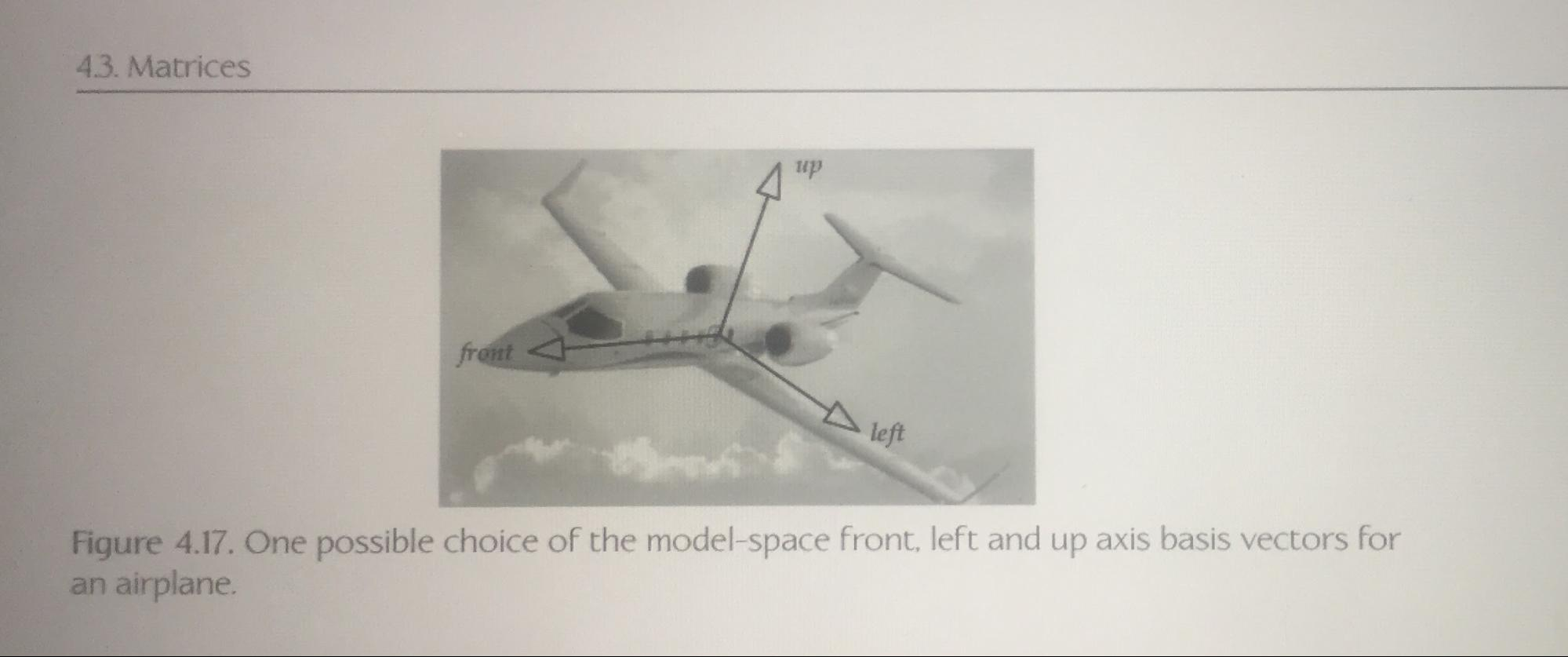### World coordinate

A word coordinate is a fixed coordinate in which position, orientation, and scale of all the elements placed in the game are described.

The origin of the coordinate system is arbitrary, but it is as a common practice to place it in the center of the world in order to reduce the number of floating point numbers needed to describe objects very far away from the origin.

The orientation of the axes is also arbitrary but usually, the convention is to have the the y-axis as the axis pointing upward.

### View space

The view space coordinates are often called as the camera coordinates. These are the coordinates fixed or attached to the game camera.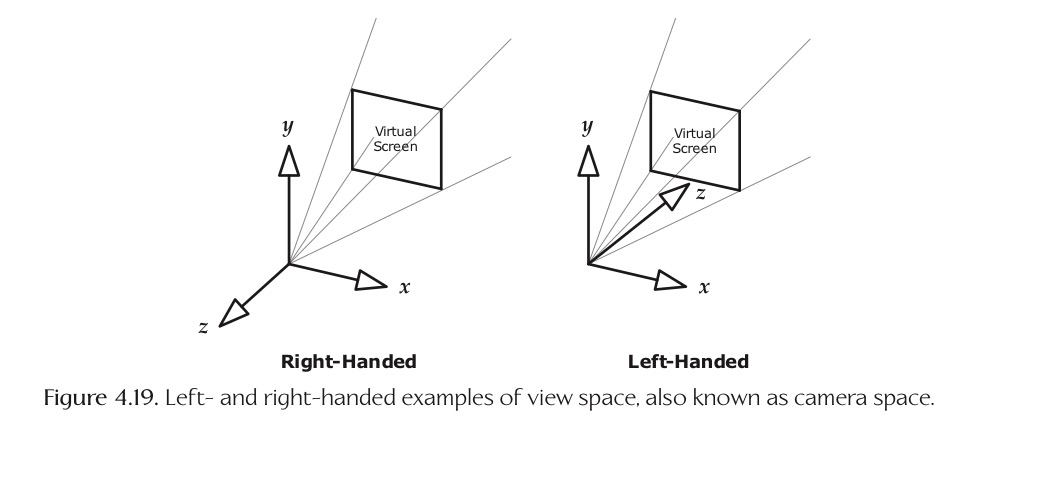As you can see from the image, you can have a right or left hand coordinate system attached to the camera.

### Coordinate space hierarchy

A coordinate space hierarchy is a coordinate system that expresses its position based on its parent object. For example, assuming you have a character with an arm attached. The vector that expresses the position of that arm is based on the coordinate system of its parent.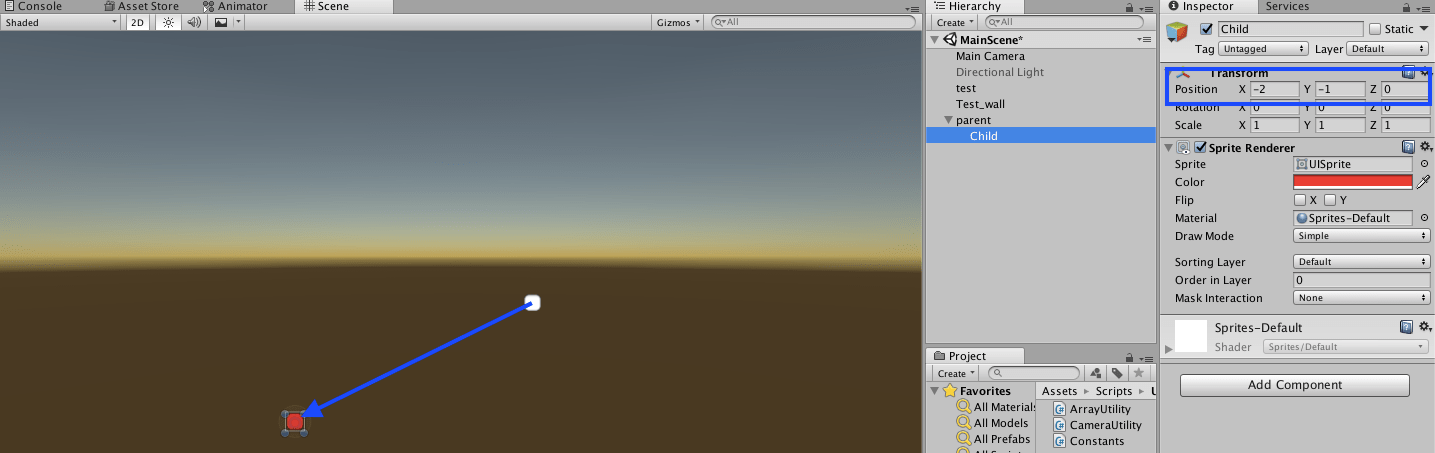As you can see from the example, the coordinates of the red box (child) are expressed as a vector from the white box (parent) position.

# Raycasting

Ray casting is a common technique used in games to find when a line (ray) has hit a specific target. A common example is when a bullet is shot from a gun.
As you can imagine, we’ll have to use Vectors to be able to correctly use the Ray Casting function available in most game engines.

### Let’s take this example from the Unity Tutorials: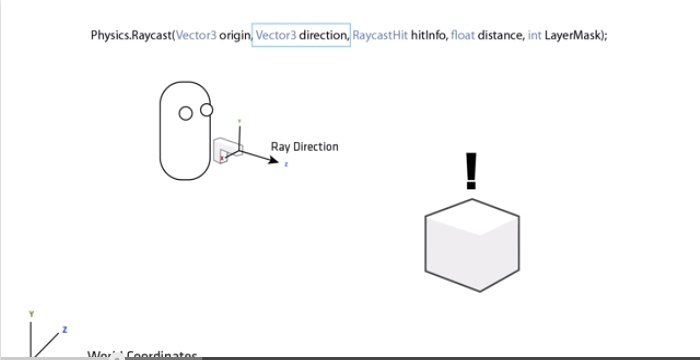Here we want to shoot a projectile and find if we have hit the target (box). As you can see, the Raycast function takes two vectors as a parameter. One is the vector “origin” which describes the origin position of the shot in the game world. The other is the ray direction, which describes where the projectile is headed.

The other parameters are not important for this lecture, but the first 2 vectors are essential to discuss as they describe the position and the direction of the shot

If you want to know more about ray casting, here is a nice tutorial.